# Single Digit Multiplication practise for year 3

Is your child familiar with multiplying single digit numbers together? Go over multiplication strategies with your child, and encourage her to do the worksheets again and beat her last time!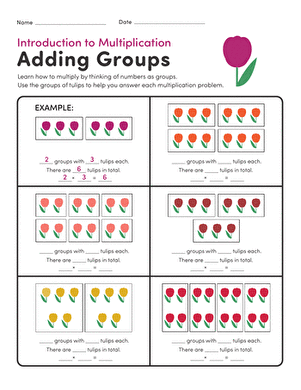### Intro to Multiplication: Adding Groups

Start your child on multiplication by practising adding groups of a certain number using flowers to help.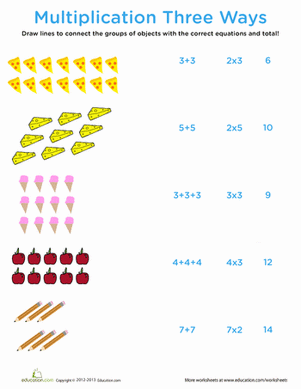### Intro to Multiplication: Multiplication Three Ways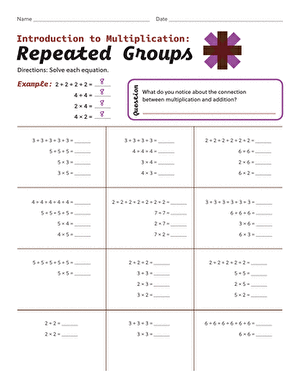### Intro to Multiplication: Repeated Groups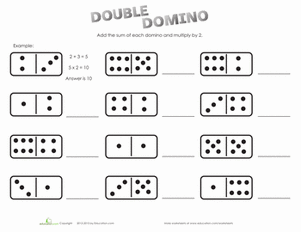### Intro to Multiplication: Multiplying by 2

Turn domino play into multiplication practise with this worksheet that helps kids learn how to multiply by 2.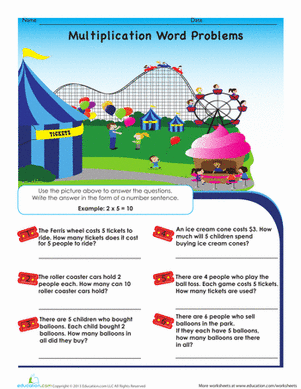### Intro to Multiplication: Roller Coaster Word Problems

Practise early multiplication skills with carnival-themed word problems packed with roller coasters, clowns, and cotton candy.### Multiply by 0

This worksheet is a great way to get your kid started on multiplication by helping him multiply by 0.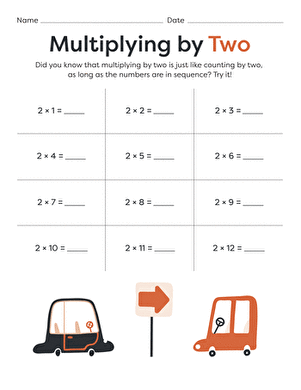### Multiplying by 2

Practise multiplying by 2 the easy way: just pretend you're counting by twos!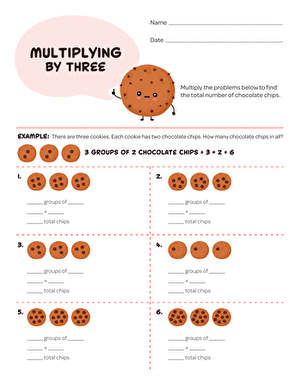### Multiplying by 3

Practise multiplying by 3 with this sweet, cookie-themed worksheet. Multiplication is a lot more fun when ice cream is involved!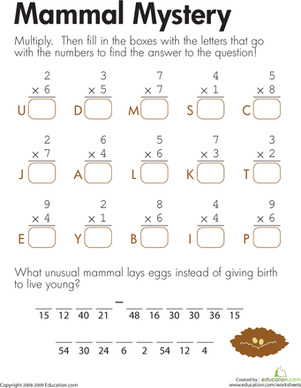### Multiplication: Mammal Mystery

Kids solve multiplication problems to crack the code and find the egg-laying mystery mammal on this year three maths worksheet.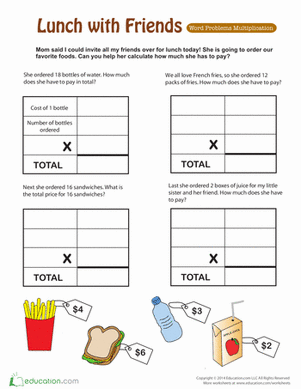### Lunch with Friends: Multiplying Money

Multiplying money word problems let your child practise his multiplication while figuring out how much lunch costs. A good way to learn the concept of money!

Create new collection

0

### New Collection>

0Items

What could we do to improve Education.com?